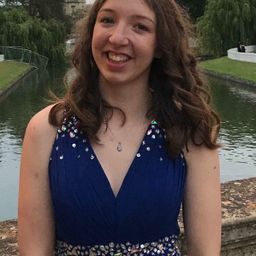### Discussion

You must be signed in to discuss.

### Video Transcript

this question is Wilma s A T Schools. Andi. I've done a rough sketch off the grass that you get given in your question. So that's got started. Part a So pop A is asking us to describe the relationship. Now the relationship is given Teoh kind of buy that line that red line there, and it shows that there's a positive relationship that it's linear or street showing by the law on you could also make a judgment of how strong it was. Now I want a long relationship would mean that the line would look almost that clear like that. Which isn't that far off on, if there was no relationship at all, really affect almost a straight line, and it's quite fall off that. So I would say that it is moderately strong, and that's that's the question. So Part B is asking us if there are any students who scores do not seem to fit the overall pattern. Now this is a lot more obvious on yoga about here. It's around the 800 on this side. Andi, it's around 500. Here you do. If you were toe drool, a dotted line down, you'd be looking about 805 100 and to me, that is a really obvious out liar. So they clearly did very well on that's in proportion how well they did on the verbal side. So I would say that there is one. Oh, and definitely make sure you label it or explain exactly where is, um, but that b B that's for that one. And then if we look a part C, it's asking us to find the correlation coefficient on. Interpret the value in this context. So our coronation competition, it's gonna be the root of all squints are all spread was given to us in the question as 46.9 so percent. The first thing you do is turn into decimal with not 0.469 and then we to square it on. This gives us a correlation coefficient with no 0.6 84 eight. That's full desperate places, um, on in terms of saying what that means In this context, that is, that's actually how correlated Matt School are verbal sat schools, and that's that looking at part Date has asked us to write the equation of the regression line, defining any variable Jews. Everything for this question is given to you in the question. So it tells you that we're trying to predict Matt schools based on verbal school. So we're looking at Ma with a hut and then, ah, intercept, which is are a is given by the intercept kerf vision. So 209 high 5542 and then our verbal sat coefficient is also given to us. Paul is here. Don't 0.6757 little, um and that's the equation you need. And in terms of defining variable, just go to say that your mouth is measured in on the points in a satin matte exam. And then verbal is the school. In the verbal of that, that's what we'd say. It's just measured in the school. So moving on to Part E. Is asking us to interpret this slope off this line of the slope Is this Butthead? It's on no 0.6757 on. That is meaning for every one extra point in verbal school we would predict arise. Yeah, off no 0.6757 points in the months, and that is what it means in the context so moving on to part s, it says, predict the mask over student with verbal school of 500. This is just some substitution. So, um, at school with a 201.542 and then we're gonna sub in that 500 the school on this here is saying to mold brothers do together, uh, talk a lot in you're going to get an answer of 547.0, no. On because we're talking to schools. It might be best around that to 547 just reading it. Fractions of points. So the last part is saying every air students calls perfect 1600 on these two parts. The test based on the model, what would be the residual? So if they score perfect 1600 we've broken at 800 on each part. So we need to look at what they were predict for someone who scored 800 on the verbal. That's what we did in the last part. But this time we're going to substitute in 100 and this is going to give us 749.61 to this is what we would predict them. But we get told that with that perfect school day to go 800 so to work out residual, we take that observed school of 800 a minus predicted school 749.6 months, which gives us a residual of 50.45 That's not the question.Other Schools